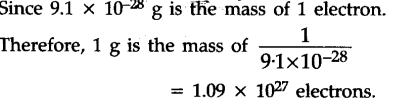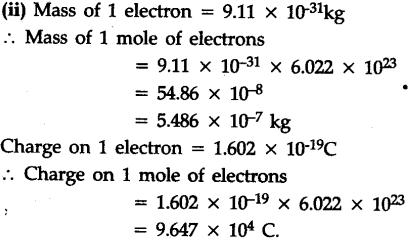# Calculate number of electrons which will together weigh one gram

(i) Calculate the number of electrons which will together weigh one gram.
(ii) Calculate the mass and^charge of one mole of electrons.
[1 mole species = 6.022 x \${{10}^{23}}\$ atom Mass of 1\${{e}^{-}}\$ = 9.11 X \${{10}^{-31}}\$ kg
Charge on 1\${{e}^{-}}\$ = 1.602 x \${{10}^{-19}}\$ C]